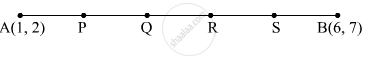Advertisement Remove all ads

# Points P, Q, R and S divides the line segment joining A(1, 2) and B(6, 7) in 5 equal parts. Find the coordinates of the points P, Q and R. - Mathematics

Answer in Brief

Points P, Q, R and S divides the line segment joining A(1, 2) and B(6, 7) in 5 equal parts. Find the coordinates of the points P, Q and R.

Advertisement Remove all ads

#### SolutionIt is given that P, Q, R and S divides the line segment joining A(1, 2) and B(6, 7) in 5 equal parts.

∴ AP = PQ = QR = RS = SB          .....(1)
Now,
AP + PQ + QR + RS + SB = AB
⇒ AP + AP + AP + AP + AP = AB             [From (1)]
⇒ 5AP = AB
⇒ AP = $\frac{1}{5}$ AB                  .....(2)

Now,
PB = PQ + QR + RS + SB = $\frac{1}{5}$ AB + $\frac{1}{5}$ AB + $\frac{1}{5}$ AB + $\frac{1}{5}$ AB =  $\frac{4}{5}$ AB         .....(3)

From (2) and (3), we get

AP : PB = $\frac{1}{5}$ AB : $\frac{4}{5}$ AB = 1 : 4
Similarly,

AQ : QB = 2 : 3 and AR : RB = 3 : 2

Using section formula, we get

Coordinates of P = $\left( \frac{1 \times 6 + 4 \times 1}{1 + 4}, \frac{1 \times 7 + 4 \times 2}{1 + 4} \right) = \left( \frac{10}{5}, \frac{15}{5} \right) = \left( 2, 3 \right)$

Coordinates of Q = $\left( \frac{2 \times 6 + 3 \times 1}{2 + 3}, \frac{2 \times 7 + 3 \times 2}{2 + 3} \right) = \left( \frac{15}{5}, \frac{20}{5} \right) = \left( 3, 4 \right)$

Coordinates of R = $\left( \frac{3 \times 6 + 2 \times 1}{3 + 2}, \frac{3 \times 7 + 2 \times 2}{3 + 2} \right) = \left( \frac{20}{5}, \frac{25}{5} \right) = \left( 4, 5 \right)$

Is there an error in this question or solution?
Advertisement Remove all ads

#### APPEARS IN

RD Sharma Class 10 Maths
Chapter 6 Co-Ordinate Geometry
Exercise 6.3 | Q 38 | Page 30
Advertisement Remove all ads

#### Video TutorialsVIEW ALL 

Advertisement Remove all ads
Share
Notifications

View all notifications

Forgot password?